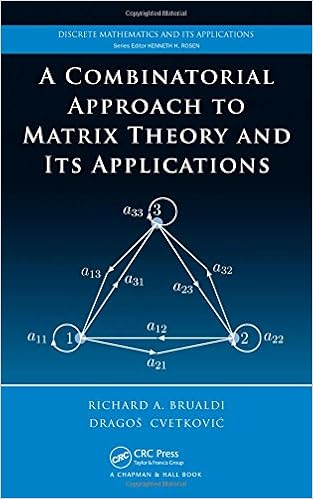# A Combinatorial Approach to Matrix Theory and Its by Richard A. BrualdiBy Richard A. Brualdi

In contrast to most simple books on matrices, A Combinatorial method of Matrix idea and Its purposes employs combinatorial and graph-theoretical instruments to enhance easy theorems of matrix conception, laying off new gentle at the topic by means of exploring the connections of those instruments to matrices.

After reviewing the fundamentals of graph concept, trouble-free counting formulation, fields, and vector areas, the ebook explains the algebra of matrices and makes use of the König digraph to hold out basic matrix operations. It then discusses matrix powers, presents a graph-theoretical definition of the determinant utilizing the Coates digraph of a matrix, and provides a graph-theoretical interpretation of matrix inverses. The authors boost the easy thought of suggestions of platforms of linear equations and exhibit tips on how to use the Coates digraph to unravel a linear process. additionally they discover the eigenvalues, eigenvectors, and attribute polynomial of a matrix; learn the \$64000 homes of nonnegative matrices which are a part of the Perron–Frobenius conception; and learn eigenvalue inclusion areas and sign-nonsingular matrices. the ultimate bankruptcy provides purposes to electric engineering, physics, and chemistry.

Using combinatorial and graph-theoretical instruments, this e-book allows a superb knowing of the basics of matrix conception and its software to medical parts.

Read or Download A Combinatorial Approach to Matrix Theory and Its Applications PDF

Similar combinatorics books

Combinatorial Pattern Matching: 17th Annual Symposium, CPM 2006, Barcelona, Spain, July 5-7, 2006. Proceedings

This e-book constitutes the refereed complaints of the seventeenth Annual Symposium on Combinatorial development Matching, CPM 2006, held in Barcelona, Spain in July 2006. The 33 revised complete papers provided including three invited talks have been conscientiously reviewed and chosen from 88 submissions. The papers are equipped in topical sections on facts buildings, indexing facts constructions, probabilistic and algebraic thoughts, purposes in molecular biology, string matching, information compression, and dynamic programming.

Algorithms in Invariant Theory

J. Kung and G. -C. Rota, of their 1984 paper, write: “Like the Arabian phoenix emerging out of its ashes, the speculation of invariants, stated lifeless on the flip of the century, is once more on the vanguard of mathematics”. The e-book of Sturmfels is either an easy-to-read textbook for invariant concept and a tough study monograph that introduces a brand new method of the algorithmic aspect of invariant thought.

Applied Combinatorics

This can be a textual content with good enough fabric for a one-semester creation to combinatorics. the unique audience was once essentially machine technology majors, however the subject matters integrated make it compatible for a number of varied scholars. subject matters comprise easy enumeration: strings, units, binomial coefficients Recursion and mathematical induction Graph thought partly ordered units extra enumeration suggestions: inclusion-exclusion, producing features, recurrence relatives, and Polya conception.

Extra info for A Combinatorial Approach to Matrix Theory and Its Applications

Sample text

In the theorem we assume that the types of the graphs and matrices are such that the operations can be carried out. 5 The following properties hold: 1. The composition of K¨onig digraphs is an associative operation: G1 ∗ (G2 ∗ G3 ) = (G1 ∗ G2 ) ∗ G3 . 2. The product of K¨onig digraphs is an associative operation: G1 · (G2 · G3 ) = (G1 · G2 ) · G3 . Equivalently, for matrices we have A1 (A2 A3 ) = (A1 A2 )A3 . 3. Graph multiplication is distributive over addition: G1 · (G2 + G3 ) = G1 · G2 + G1 · G3 (G1 + G2 ) · G3 = G1 · G3 + G2 · G3 .

U(m) ). A spanning set S of V is a minimal spanning set of V provided that each set of vectors obtained from S by removing a vector is not a spanning set for V . A linearly independent set S of vectors of V is a maximal linearly independent set of vectors of V provided that for each vector w of V that is not in S, S ∪ {w} is linearly dependent (when this happens, w must be a linear combination of the vectors in S). ✷ In the next theorem, we collect some basic facts about these properties. 2 Let V be a subspace of F n .

THE KONIG DIGRAPH OF A MATRIX 41 The anticommutativity property (AB)T = B T AT can be understood in terms of the K¨onig digraph. Consider the composition digraphs G(A) ∗ G(B) and G(B T ) ∗ G(AT ). If in G(A) ∗ G(B) we make the black vertices white and make the white vertices black and change the orientation of all edges, then we get the digraph G(B T ) ∗ G(AT ). This implies that (AB)T = B T AT . Finally we note that using induction we get the more general producttransposition rule: (A1 A2 · · · Ak )T = ATk · · · AT2 AT1 (k ≥ 2).

Download PDF sample

Rated 4.29 of 5 – based on 42 votes